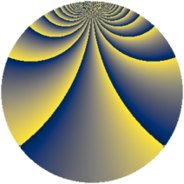# Properties

 Label 1323.1.bvLevel $1323$ Weight $1$ Character orbit 1323.bv Rep. character $\chi_{1323}(82,\cdot)$ Character field $\Q(\zeta_{42})$ Dimension $12$ Newform subspaces $1$ Sturm bound $168$ Trace bound $0$

# Related objects

## Defining parameters

 Level: $$N$$ $$=$$ $$1323 = 3^{3} \cdot 7^{2}$$ Weight: $$k$$ $$=$$ $$1$$ Character orbit: $$[\chi]$$ $$=$$ 1323.bv (of order $$42$$ and degree $$12$$) Character conductor: $$\operatorname{cond}(\chi)$$ $$=$$ $$49$$ Character field: $$\Q(\zeta_{42})$$ Newform subspaces: $$1$$ Sturm bound: $$168$$ Trace bound: $$0$$

## Dimensions

The following table gives the dimensions of various subspaces of $$M_{1}(1323, [\chi])$$.

Total New Old
Modular forms 84 12 72
Cusp forms 12 12 0
Eisenstein series 72 0 72

The following table gives the dimensions of subspaces with specified projective image type.

$$D_n$$ $$A_4$$ $$S_4$$ $$A_5$$
Dimension 12 0 0 0

## Trace form

 $$12 q - q^{4} + 2 q^{7} + O(q^{10})$$ $$12 q - q^{4} + 2 q^{7} + q^{16} + q^{25} + q^{28} + 3 q^{31} - 13 q^{37} - 2 q^{43} - 2 q^{49} + 4 q^{52} + 4 q^{61} + 2 q^{64} - q^{67} - q^{79} + O(q^{100})$$

## Decomposition of $$S_{1}^{\mathrm{new}}(1323, [\chi])$$ into newform subspaces

Label Dim $A$ Field Image CM RM Traces $q$-expansion
$a_{2}$ $a_{3}$ $a_{5}$ $a_{7}$
1323.1.bv.a $12$ $0.660$ $$\Q(\zeta_{21})$$ $D_{42}$ $$\Q(\sqrt{-3})$$ None $$0$$ $$0$$ $$0$$ $$2$$ $$q-\zeta_{42}^{10}q^{4}-\zeta_{42}^{12}q^{7}+(\zeta_{42}^{4}-\zeta_{42}^{20}+\cdots)q^{13}+\cdots$$